# Transistor_Biasing.pdf

Mar. 25, 2023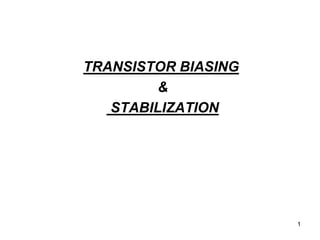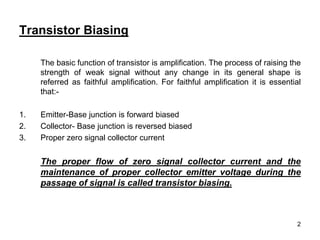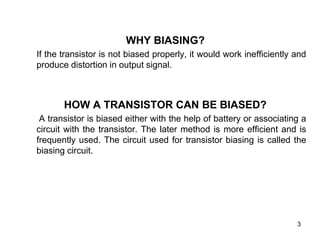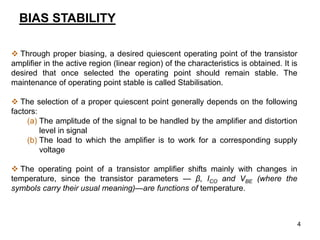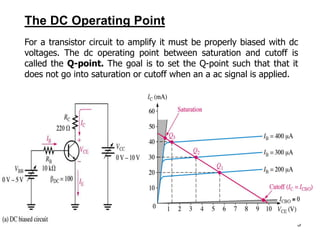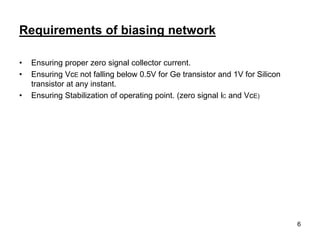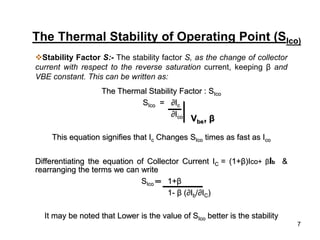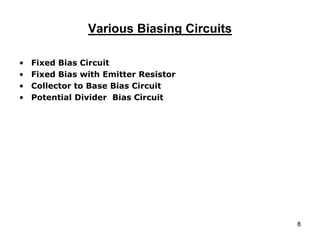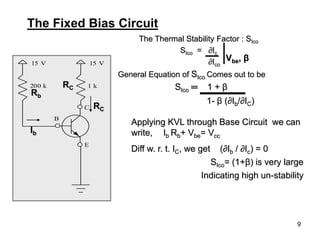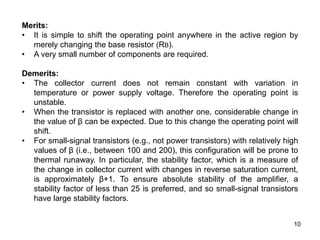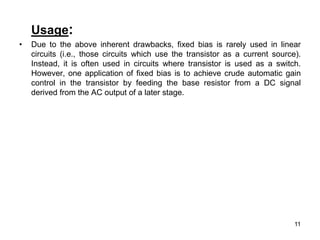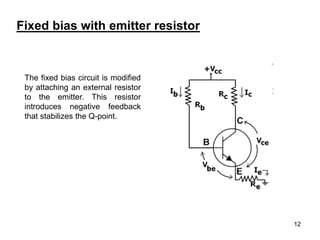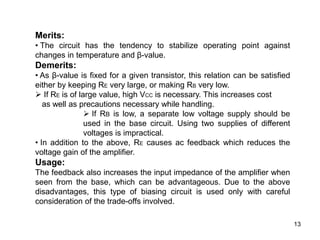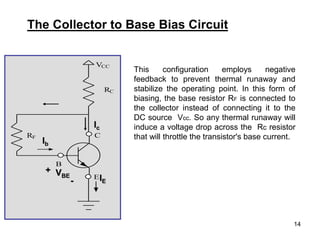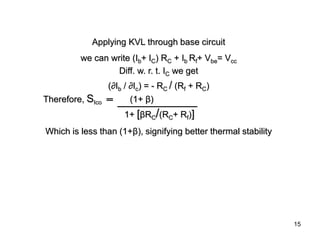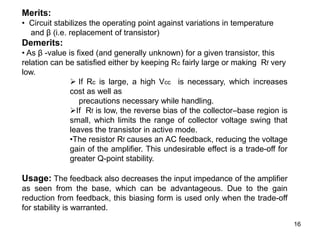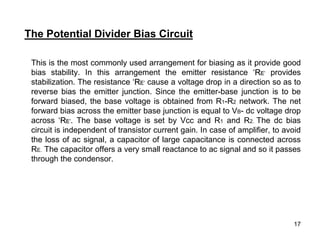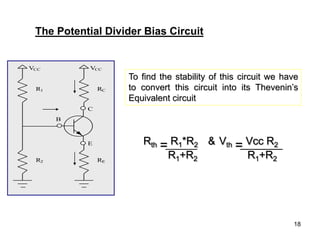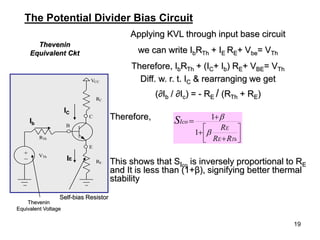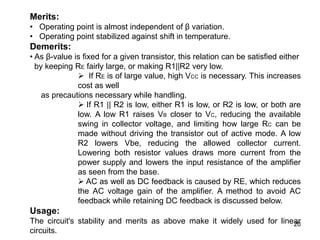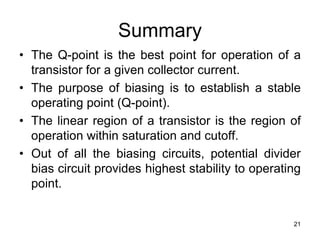1 of 22

### Transistor_Biasing.pdf

1. 1 TRANSISTOR BIASING & STABILIZATION
2. 2 Transistor Biasing The basic function of transistor is amplification. The process of raising the strength of weak signal without any change in its general shape is referred as faithful amplification. For faithful amplification it is essential that:- 1. Emitter-Base junction is forward biased 2. Collector- Base junction is reversed biased 3. Proper zero signal collector current The proper flow of zero signal collector current and the maintenance of proper collector emitter voltage during the passage of signal is called transistor biasing.
3. 3 WHY BIASING? If the transistor is not biased properly, it would work inefficiently and produce distortion in output signal. HOW A TRANSISTOR CAN BE BIASED? A transistor is biased either with the help of battery or associating a circuit with the transistor. The later method is more efficient and is frequently used. The circuit used for transistor biasing is called the biasing circuit.
4. 4 BIAS STABILITY  Through proper biasing, a desired quiescent operating point of the transistor amplifier in the active region (linear region) of the characteristics is obtained. It is desired that once selected the operating point should remain stable. The maintenance of operating point stable is called Stabilisation.  The selection of a proper quiescent point generally depends on the following factors: (a) The amplitude of the signal to be handled by the amplifier and distortion level in signal (b) The load to which the amplifier is to work for a corresponding supply voltage  The operating point of a transistor amplifier shifts mainly with changes in temperature, since the transistor parameters — β, ICO and VBE (where the symbols carry their usual meaning)—are functions of temperature.
5. 5 The DC Operating Point For a transistor circuit to amplify it must be properly biased with dc voltages. The dc operating point between saturation and cutoff is called the Q-point. The goal is to set the Q-point such that that it does not go into saturation or cutoff when an a ac signal is applied.
6. 6 Requirements of biasing network • Ensuring proper zero signal collector current. • Ensuring VcE not falling below 0.5V for Ge transistor and 1V for Silicon transistor at any instant. • Ensuring Stabilization of operating point. (zero signal IC and VcE)
7. 7 The Thermal Stability of Operating Point (SIco) Stability Factor S:- The stability factor S, as the change of collector current with respect to the reverse saturation current, keeping β and VBE constant. This can be written as: The Thermal Stability Factor : SIco SIco = ∂Ic ∂Ico This equation signifies that Ic Changes SIco times as fast as Ico Differentiating the equation of Collector Current IC = (1+β)Ico+ βIb & rearranging the terms we can write SIco ═ 1+β 1- β (∂Ib/∂IC) It may be noted that Lower is the value of SIco better is the stability Vbe, β
8. 8 Various Biasing Circuits • Fixed Bias Circuit • Fixed Bias with Emitter Resistor • Collector to Base Bias Circuit • Potential Divider Bias Circuit
9. 9 The Fixed Bias Circuit 15 V C E B 15 V 200 k 1 k The Thermal Stability Factor : SIco SIco = ∂Ic ∂Ico General Equation of SIco Comes out to be SIco ═ 1 + β 1- β (∂Ib/∂IC) Vbe, β Applying KVL through Base Circuit we can write, Ib Rb+ Vbe= Vcc Diff w. r. t. IC, we get (∂Ib / ∂Ic) = 0 SIco= (1+β) is very large Indicating high un-stability Ib Rb RC RC
10. 10 Merits: • It is simple to shift the operating point anywhere in the active region by merely changing the base resistor (RB). • A very small number of components are required. Demerits: • The collector current does not remain constant with variation in temperature or power supply voltage. Therefore the operating point is unstable. • When the transistor is replaced with another one, considerable change in the value of β can be expected. Due to this change the operating point will shift. • For small-signal transistors (e.g., not power transistors) with relatively high values of β (i.e., between 100 and 200), this configuration will be prone to thermal runaway. In particular, the stability factor, which is a measure of the change in collector current with changes in reverse saturation current, is approximately β+1. To ensure absolute stability of the amplifier, a stability factor of less than 25 is preferred, and so small-signal transistors have large stability factors.
11. 11 Usage: • Due to the above inherent drawbacks, fixed bias is rarely used in linear circuits (i.e., those circuits which use the transistor as a current source). Instead, it is often used in circuits where transistor is used as a switch. However, one application of fixed bias is to achieve crude automatic gain control in the transistor by feeding the base resistor from a DC signal derived from the AC output of a later stage.
12. 12 The fixed bias circuit is modified by attaching an external resistor to the emitter. This resistor introduces negative feedback that stabilizes the Q-point. Fixed bias with emitter resistor
13. 13 Merits: • The circuit has the tendency to stabilize operating point against changes in temperature and β-value. Demerits: • As β-value is fixed for a given transistor, this relation can be satisfied either by keeping RE very large, or making RB very low.  If RE is of large value, high VCC is necessary. This increases cost as well as precautions necessary while handling.  If RB is low, a separate low voltage supply should be used in the base circuit. Using two supplies of different voltages is impractical. • In addition to the above, RE causes ac feedback which reduces the voltage gain of the amplifier. Usage: The feedback also increases the input impedance of the amplifier when seen from the base, which can be advantageous. Due to the above disadvantages, this type of biasing circuit is used only with careful consideration of the trade-offs involved.
14. 14 The Collector to Base Bias Circuit VCC RC C E B RF Ic Ib VBE + - IE This configuration employs negative feedback to prevent thermal runaway and stabilize the operating point. In this form of biasing, the base resistor RF is connected to the collector instead of connecting it to the DC source Vcc. So any thermal runaway will induce a voltage drop across the Rc resistor that will throttle the transistor's base current.
15. 15 Applying KVL through base circuit we can write (Ib+ IC) RC + Ib Rf+ Vbe= Vcc Diff. w. r. t. IC we get (∂Ib / ∂Ic) = - RC / (Rf + RC) Therefore, SIco ═ (1+ β) 1+ [βRC/(RC+ Rf)] Which is less than (1+β), signifying better thermal stability
16. 16 Merits: • Circuit stabilizes the operating point against variations in temperature and β (i.e. replacement of transistor) Demerits: • As β -value is fixed (and generally unknown) for a given transistor, this relation can be satisfied either by keeping Rc fairly large or making Rf very low.  If Rc is large, a high Vcc is necessary, which increases cost as well as precautions necessary while handling. If Rf is low, the reverse bias of the collector–base region is small, which limits the range of collector voltage swing that leaves the transistor in active mode. •The resistor Rf causes an AC feedback, reducing the voltage gain of the amplifier. This undesirable effect is a trade-off for greater Q-point stability. Usage: The feedback also decreases the input impedance of the amplifier as seen from the base, which can be advantageous. Due to the gain reduction from feedback, this biasing form is used only when the trade-off for stability is warranted.
17. 17 This is the most commonly used arrangement for biasing as it provide good bias stability. In this arrangement the emitter resistance ‘RE’ provides stabilization. The resistance ‘RE’ cause a voltage drop in a direction so as to reverse bias the emitter junction. Since the emitter-base junction is to be forward biased, the base voltage is obtained from R1-R2 network. The net forward bias across the emitter base junction is equal to VB- dc voltage drop across ‘RE’. The base voltage is set by Vcc and R1 and R2. The dc bias circuit is independent of transistor current gain. In case of amplifier, to avoid the loss of ac signal, a capacitor of large capacitance is connected across RE. The capacitor offers a very small reactance to ac signal and so it passes through the condensor. The Potential Divider Bias Circuit
18. 18 VCC RC C E B VCC R1 RE R2 IE IC Ib The Potential Divider Bias Circuit To find the stability of this circuit we have to convert this circuit into its Thevenin’s Equivalent circuit Rth = R1*R2 & Vth = Vcc R2 R1+R2 R1+R2 VCC RC C E B VCC R1 RE R2 Rth = R1*R2 & Vth = Vcc R2 R1+R2 R1+R2 Rth = R1*R2 & Vth = Vcc R2 R1+R2 R1+R2 Rth = R1*R2 & Vth = Vcc R2 R1+R2 R1+R2
19. 19 Applying KVL through input base circuit we can write IbRTh + IE RE+ Vbe= VTh Therefore, IbRTh + (IC+ Ib) RE+ VBE= VTh Diff. w. r. t. IC & rearranging we get (∂Ib / ∂Ic) = - RE / (RTh + RE) Therefore, This shows that SIco is inversely proportional to RE and It is less than (1+β), signifying better thermal stability Thevenin Equivalent Ckt           Th R R R E E Ico S   1 1 The Potential Divider Bias Circuit VCC RC C E B RE RTh VTh _ + Thevenin Equivalent Voltage Self-bias Resistor IE Ib IC
20. 20 Merits: • Operating point is almost independent of β variation. • Operating point stabilized against shift in temperature. Demerits: • As β-value is fixed for a given transistor, this relation can be satisfied either by keeping RE fairly large, or making R1||R2 very low.  If RE is of large value, high VCC is necessary. This increases cost as well as precautions necessary while handling.  If R1 || R2 is low, either R1 is low, or R2 is low, or both are low. A low R1 raises VB closer to VC, reducing the available swing in collector voltage, and limiting how large RC can be made without driving the transistor out of active mode. A low R2 lowers Vbe, reducing the allowed collector current. Lowering both resistor values draws more current from the power supply and lowers the input resistance of the amplifier as seen from the base.  AC as well as DC feedback is caused by RE, which reduces the AC voltage gain of the amplifier. A method to avoid AC feedback while retaining DC feedback is discussed below. Usage: The circuit's stability and merits as above make it widely used for linear circuits.
21. 21 Summary • The Q-point is the best point for operation of a transistor for a given collector current. • The purpose of biasing is to establish a stable operating point (Q-point). • The linear region of a transistor is the region of operation within saturation and cutoff. • Out of all the biasing circuits, potential divider bias circuit provides highest stability to operating point.
22. 22 THANK YOU